## Add space between number and text in a cell in Excel

In this article we will learn how to add space between number and text with formulas in Excel.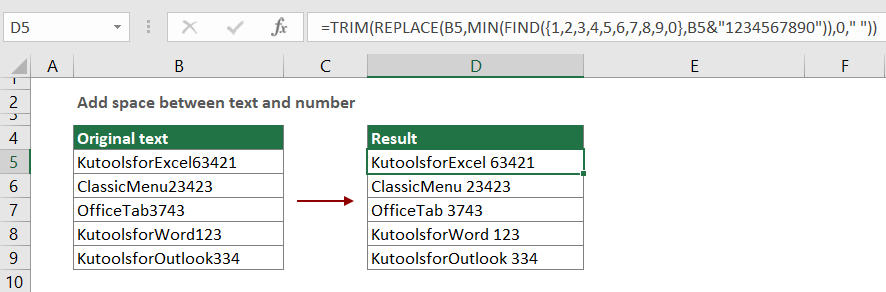Case 1: Add space between number and text – the text always comes first
Case 2: Add space between number and text – the number always comes first

#### Add space between number and text – the text always comes first

Supposing there is a text string list contains number and text where the text always comes first as the below screenshot shown, you can add space between the number and text with the below formula.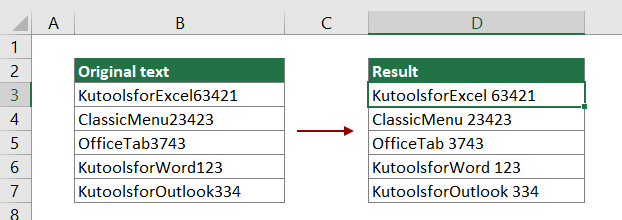Generic formula

=TRIM(REPLACE(text_string,MIN(FIND({1,2,3,4,5,6,7,8,9,0},text_string&”1234567890”)),0,” “))

Arguments

Text_string: The text string you will add space between the text and number. It can be:

• The text string enclosed in quotation marks;
• Or a reference to a cell containing the text string.

How to use this formula?

1. Select a blank cell to output the result. In this case, I select D3.

2. Enter the below formula into it and press the Enter key. And then Drag this result cell all the way down to apply the formula to other cells.

=TRIM(REPLACE(B3,MIN(FIND({1,2,3,4,5,6,7,8,9,0},B3&"1234567890")),0," "))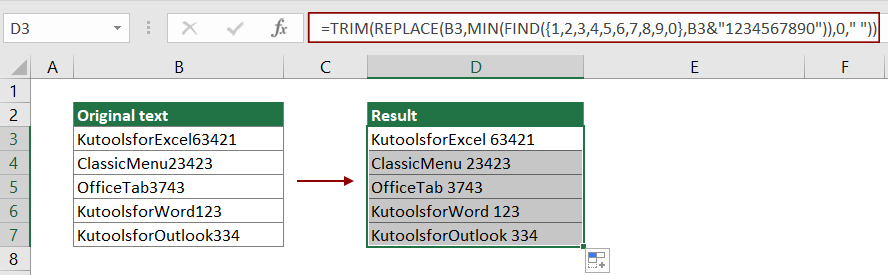Notes:

• In the formula, B3 is the text string cell which you will add space between the text and number;
• This formula can only add a space before the first number. For example, this formula will change B34C12G45 to B 34C12G45.

How this formula works?

=TRIM(REPLACE(B3,MIN(FIND({1,2,3,4,5,6,7,8,9,0},B3&"1234567890")),0," "))

1. FIND({1,2,3,4,5,6,7,8,9,0},B3&"1234567890")

• B3&"1234567890": returns the result as: "KutoolsforExcel634211234567890";
• FIND({1,2,3,4,5,6,7,8,9,0}, "KutoolsforExcel634211234567890"): The FIND function finds the position of "1-0" digits in KutoolsforExcel634211234567890 and returns the result as {20,19,17,18,25,16,27,28,29,30}.
Note: The result {20,19,17,18,25,16,27,28,29,30} means that the number 1 is in the 20th position in KutoolsforExcel634211234567890, number 2 is in the 19th position in KutoolsforExcel634211234567890…

2. MIN({20,19,17,18,25,16,27,28,29,30})

• The MIN function gets the minimum value of the array. Here the result is 16.

3. REPLACE(KutoolsforExcel63421,16,0," ")

• Here use the REPLACE function to add a space to the 16th position in KutoolsforExcel63421 and return the result as KutoolsforExcel 63421.

4. =TRIM("KutoolsforExcel 63421")

• The TRIM function Removes all spaces from text except for single spaces between words. And keeps no leading and trailing spaces of the text.

#### Add space between number and text – the number always comes first

Another case as the below screenshot shown, there is a text string list contains number and text where the number always comes first, you can apply the below array formula to add a space between the number and text.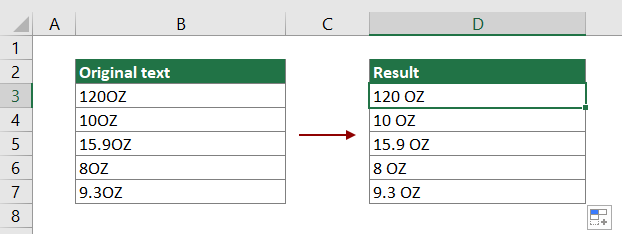Generic formula

=TRIM(REPLACE(text_string,MAX(IFERROR(FIND({0,1,2,3,4,5,6,7,8,9},text_string,ROW(INDIRECT("1:"&LEN(text_string)))),0))+1,0," ")) + Ctrl + Shift + Enter

Arguments

Text_string: The text string you will add space between the text and number. It can be:

• The text string enclosed in quotation marks;
• Or a reference to a cell containing the text string.

How to use this formula?

1. Select a blank cell (D3 in this case) to output the result.

2. Enter the below formula into it and then press the Ctrl + Shift + Enter keys simultaneously.

=TRIM(REPLACE(B3,MAX(IFERROR(FIND({0,1,2,3,4,5,6,7,8,9},B3,ROW(INDIRECT("1:"&LEN(B3)))),0))+1,0," "))

3. Select the result cell, and then drag it all the way down to apply the formula to other cells as the below screenshot shown.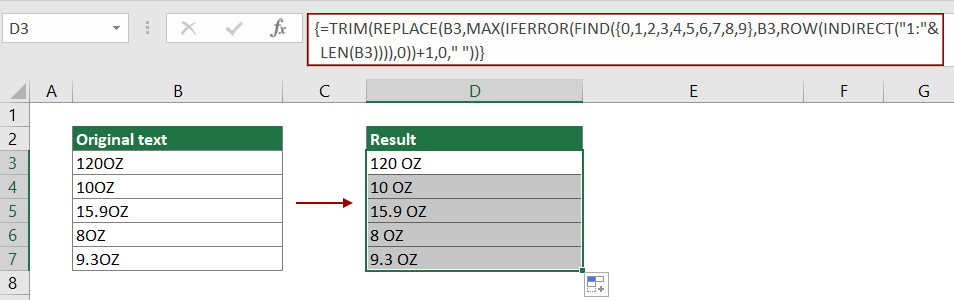Notes:

• In the formula, B3 is the text string cell which you will add space between the text and number;
• This array formula can only add a space after the last number. For example, this formula will change 34C12GHH to 34C12 GHH.

#### Related functions

Excel TRIM function
The Excel TRIM function removes all extra spaces from text string and only keeps single spaces between words.

Excel REPLACE function
The Excel REPLACE function finds and replaces characters based on given location from text string with a new text.

Excel MIN function
The Excel MIN function returns the smallest number in a set of values.

Excel FIND function
The Excel FIND function is used to find a string within another string, and returns the starting position of the string inside another one.

Excel MAX function
The Excel MAX function returns the largest number in a set of values.

Excel IFERROR function
The IFERROR function is used to return a custom result when a formula evaluates an error, and return a normal result when no error is occurred.

Excel ROW function
The Excel ROW function returns the row number of a reference.

Excel INDIRECT function
The Excel INDIRECT function converts a text string to a valid reference.

Excel LEN function
The Excel LEN function returns the number of characters in a text string.

#### Related formulas

Add comma after first word in a cell in Excel
To add a comma after the first word only in a cell, the formula in this tutorial can do you a favor.

Add a specific character before each word in a cell
This article explains how to add a specific character before each word in a cell with formula in Excel.

This article explains how to add dashes to phone number in a certain cell with formula in Excel.

To add comma between names in a cell, the formula in this tutorial can do you a favor.

### The Best Office Productivity Tools

#### Kutools for Excel - Helps You To Stand Out From Crowd

Would you like to complete your daily work quickly and perfectly? Kutools for Excel brings 300 powerful advanced features (Combine workbooks, sum by color, split cell contents, convert date, and so on...) and save 80% time for you.

• Designed for 1500 work scenarios, helps you solve 80% Excel problems.
• Reduce thousands of keyboard and mouse clicks every day, relieve your tired eyes and hands.
• Become an Excel expert in 3 minutes. No longer need to remember any painful formulas and VBA codes.
• 30-day unlimited free trial. 60-day money back guarantee. Free upgrade and support for 2 years.#### Office Tab - Enable Tabbed Reading and Editing in Microsoft Office (include Excel)

• One second to switch between dozens of open documents!
• Reduce hundreds of mouse clicks for you every day, say goodbye to mouse hand.
• Increases your productivity by 50% when viewing and editing multiple documents.
• Brings Efficient Tabs to Office (include Excel), Just Like Chrome, Firefox, And New Internet Explorer.